# NCERT Solutions for Class 7 Maths Chapter 3 Data Handling

NCERT Solutions for Class 7 Maths Chapter 3 Data Handling

### NCERT Solutions for Class 7 Maths Chapter 3 Data Handling Ex 3.1

NCERT Solutions for Class 7 Maths Chapter 3 Data Handling Exercise 3.1
Ex 3.1 Class 7 Maths Question 1.
Find the range of heights of any ten students of your class.
Solution:
Let us have the heights of 10 students are as follows:
140 cm, 141.5 cm, 138 cm, 150 cm, 161 cm,
138 cm, 140.5 cm, 135.5 cm, 160 cm, 158 cm
Here, minimum height = 135.5 cm
Maximum height =161 cm
∴ Range = Maximum height – Minimum height
= 161 cm — 135.5 cm = 25.5 cm
Hence, the required range = 25.5 cm.

Ex 3.1 Class 7 Maths Question 2.
Organise the following marks in a class assessment in a tabular form.
4, 6, 7, 5, 3, 5, 4, 5, 2, 6, 2, 5, 1, 9, 6, 5, 8, 4, 6, 7
(i) Which number is the highest?
(ii) Which number is the lowest?
(iii) What is the range of the data?
(iv) Find the arithmetic mean.
Solution:
Let us form a frequency distribution table: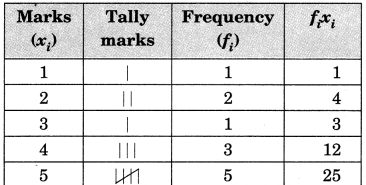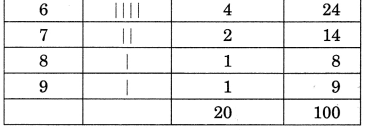(i) 9 is the highest marks.
(ii) 1 is the lowest marks.
(iii) Range = Max. marks – Min. marks
= 9 – 1 = 8Ex 3.1 Class 7 Maths Question 3.
Find the mean of first five whole numbers.
Solution:Ex 3.1 Class 7 Maths Question 4.
A cricketer scores the following runs in eight innings:
58, 76, 40, 35, 46, 45, 0, 100
Find the mean score.
Solution:
Following are the scores of the runs in eight innings:
58, 76, 40, 35, 46, 45, 0, 100Ex 3.1 Class 7 Maths Question 5.
Following table shows the points of each player scored in four games:

 Player Game 1 Game 2 Game 3 Game 4 A 14 16 10 10 B 0 8 6 4 C 8 11 Did not play 13

(i) Find the mean to determine A’s average number of points scored per game.
(ii) To find the mean number of points per game for C, would you divide the total points by 3 or by 4? Why?
(iii) B played in all the four games. How would you find the mean?
(iv) Who is the best performer?
Solution:
(i) Number of points scored by A in all games are
Game 1 – 14, Game 2 = 16, Game 3 = 10, Game 4 = 10Ex 3.1 Class 7 Maths Question 6.
The marks (out of 100) obtained by a group of students in a science test are 85, 76, 90, 85, 39, 48, 56, 95, 81 and 75. Find the
(i) highest and the lowest marks obtained by the students.
(ii) range of the marks obtained.
(iii) mean marks obtained by the group.
Solution:
Marks obtained are:
85, 76, 90, 85, 39, 48, 56, 95, 81 and 75
(i) Highest marks = 95
Lowest marks = 39
(ii) Range of the marks
= Highest marks – Lowest marks
= 95 – 39 = 56
(iii) Mean marks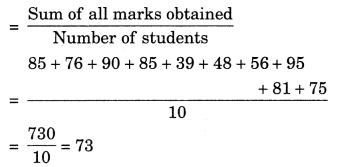Ex 3.1 Class 7 Maths Question 7.
The enrolment in a school during six consecutive years was as follows:
1555, 1670, 1750, 2013, 2540, 2820
Find the mean enrolment of the school for this period.
Solution:
Mean enrolment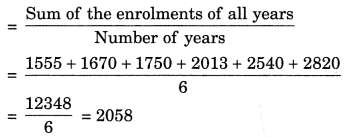Thus, the required mean = 2058.

Ex 3.1 Class 7 Maths Question 8.
The rainfall (in mm) in a city on 7 days of a certain week was recorded as follows:

 Day Rainfall (in mm) Monday 0.0 Tuesday 12.2 Wednesday 2.1 Thursday 0.0 Friday 20.5 Saturday 5.5 Sunday 1.0

(i) Find the range of the rainfall in the above data.
(ii) Find the mean rainfall for the week.
(iii) On how many days was the rainfall less than the mean rainfall?
Solution:
(i) Maximum rainfall = 20.5 mm
Minimum rainfall = 0.0 mm
∴ Range = Maximum rainfall – Minimum rainfall
= 20.5 mm – 0.0 mm = 20.5 mm

(ii) Mean rainfall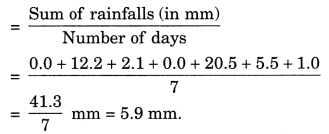(iii) Number of days on which the rainfall was less than the mean rainfall = Monday, Wednesday, Thursday, Saturday, Sunday = 5 days.

Ex 3.1 Class 7 Maths Question 9.
The heights of 10 girls were measured in cm and the results are as follows:
135, 150, 139, 128, 151, 132, 146, 149, 143, 141
(i) What is the height of the tallest girl?
(ii) What is the height of the shortest girl?
(Hi) What is the range of the data?
(iv) What is the mean height of the girls?
(v) How many girls have heights more than the mean height?
Solution:
(i) Height of the tallest girl = 151 cm.
(ii) Height of the shortest girl = 128 cm.
(iii) Range = Height of tallest girl – Height of the shortest girl
= 151 cm – 128 cm = 23 cm.(v) Number of girls having more height than the mean height
= 150, 151, 146, 149 and 143 = 5 girls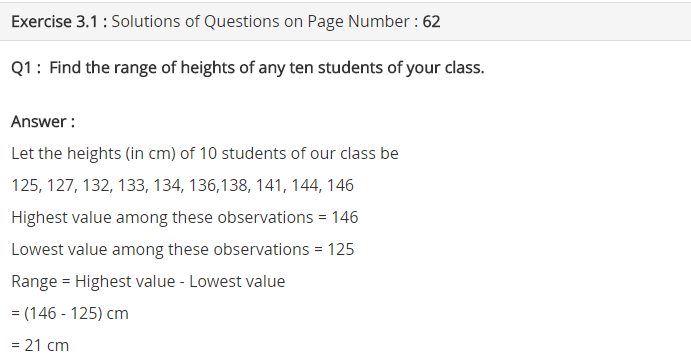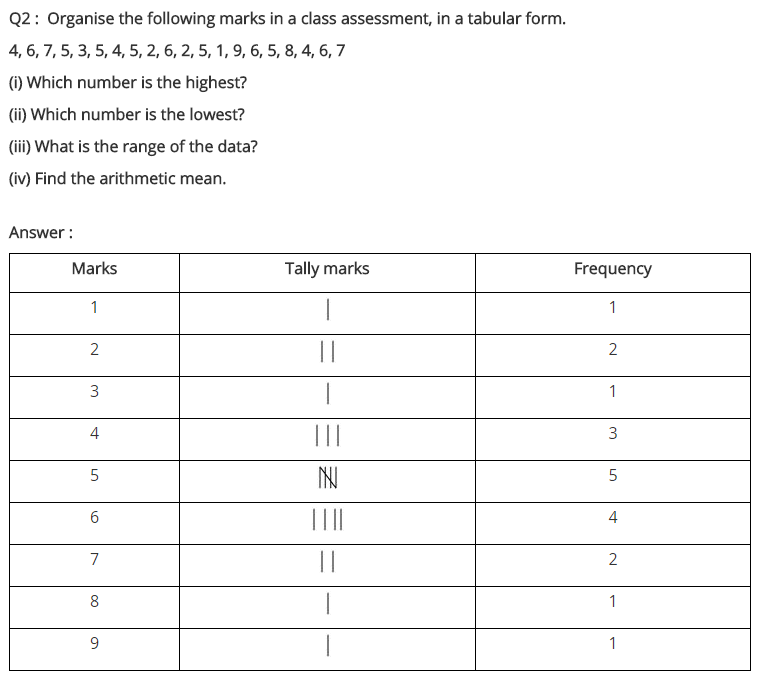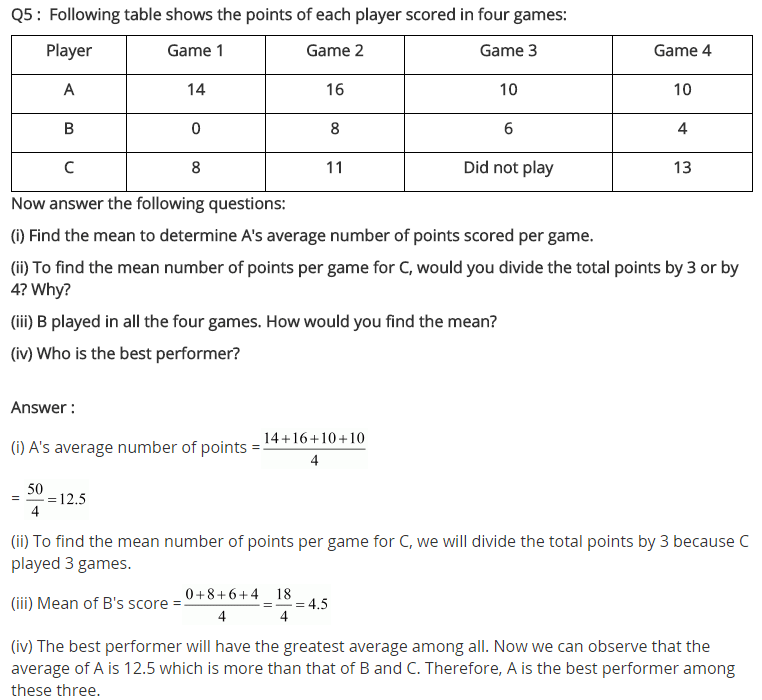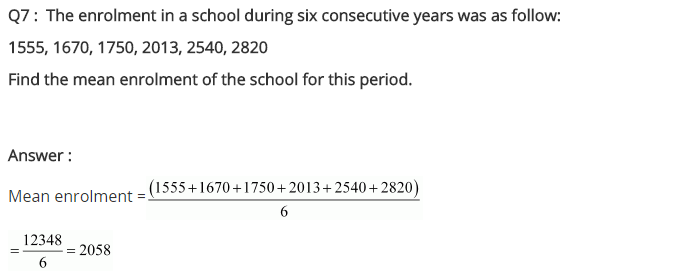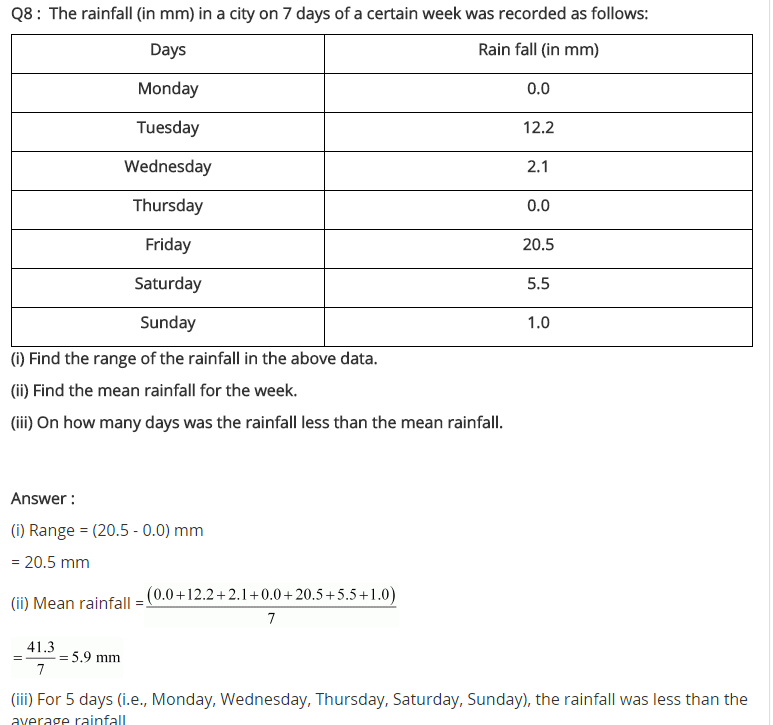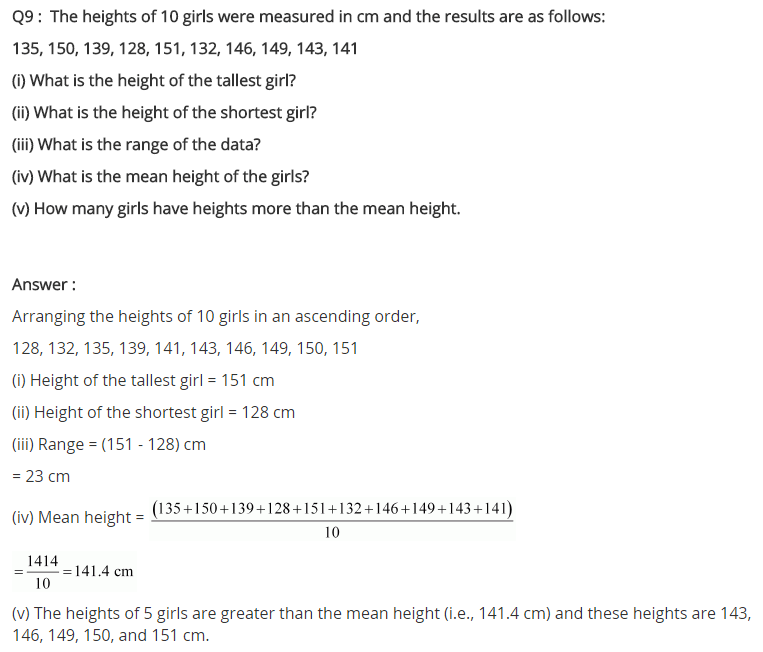## SabDekho

The Complete Educational Website